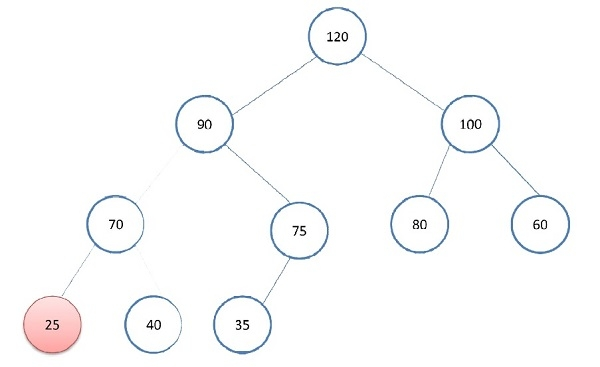# Minimum element in a max heap in C++.

## Problem statement

Find the element with the least value in max heap.

Let us consider below max heap.In max heap value of the root node is always greater than its childer. Because of this property, we can conclude that value will be present in one of the leaf nodes. If heap contains n nodes then there will be ceil(n/2) leaves.

Max heap is a complete binary tree hence it can be represented in an array. In such heap the first leaf will be present after floor(n/2) index. So in our example, the first leave will be present at index 5.

## Algorithm

We can use the below algorithm to find min value in a max heap −

1. Find first leaf in a heap and consider its value as min
2. Iterate all remaining leaves and update min value if leaf with smaller value is found

## Example

#include <iostream>
#define SIZE(arr) (sizeof(arr) / sizeof(arr))
using namespace std;
int getMinElement(int *heap, int n){
int minElement = heap[n / 2];
for (int i = n / 2 + 1; i < n; ++i) {
minElement = min(minElement, heap[i]);
}
return minElement;
}
int main(){
int heap[] = {120, 90, 100, 70, 75, 80, 60, 25, 40, 35};
cout << "Min value: " << getMinElement(heap, SIZE(heap)) << "\n";
return 0;
}

## Output

When you compile and execute the above program. It generates the following output −

Min value: 25